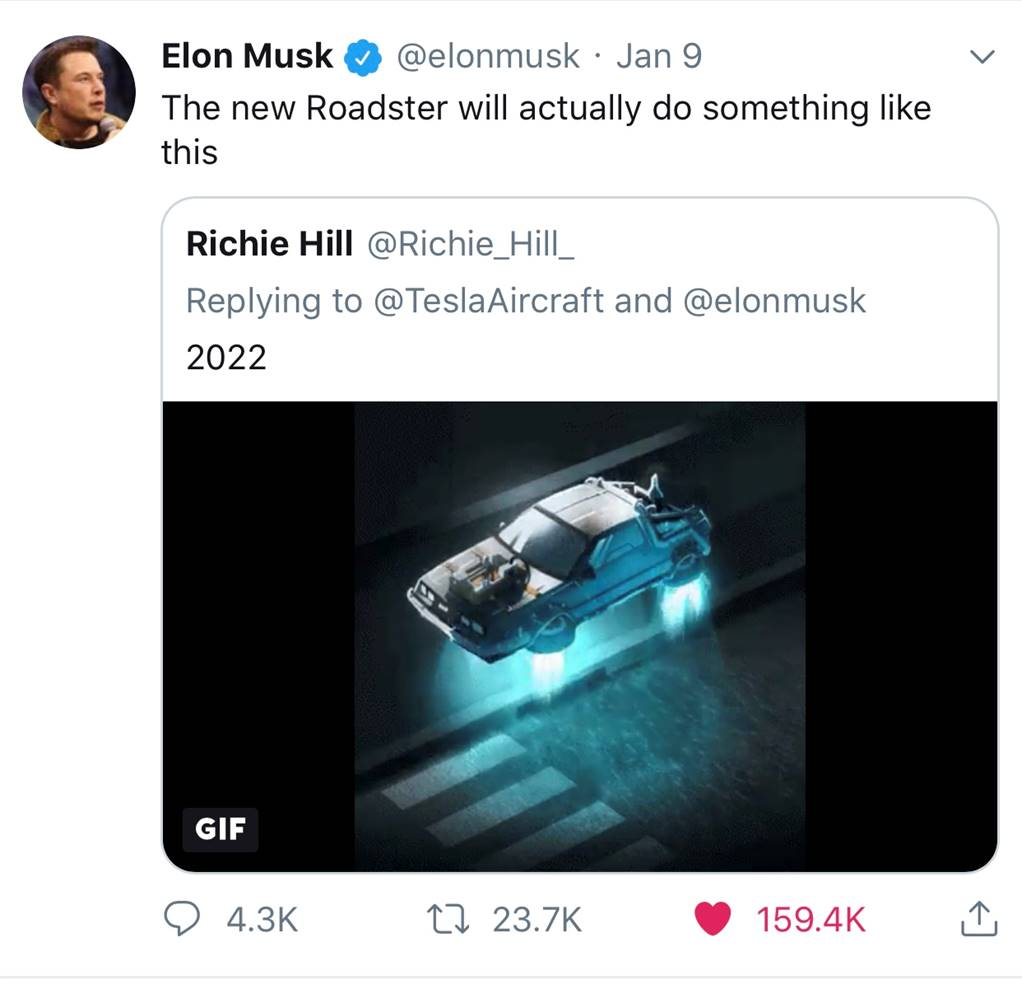Can SpaceX Really Make a Tesla Fly?Following the unveil of Tesla’s super-fast new roadster a year ago, Elon Musk tweeted that it was just the base model, promising a ‘SpaceX’ version with much enhanced performance. He then upped the ante by saying that the SpaceX technology might allow the car to fly. Many assumed he was joking, but it seems not – he just tweeted the claim again, with a clip of a hovering DeLorean.

But is this even possible? Could SpaceX technology really make a Tesla Roadster fly?

(Well, apart from on the end of Falcon Heavy)

There’s been a lot of (excuse the pun) hot air written about this, including empty opinions from car industry pundits. But opinion is not required. The question is really just a basic Physics and rocketry problem. So I thought I’d whip out my digital envelope and scrawl some calculations on the back to see if I could get a more quantitive answer. Here goes …

First of all, what does Elon mean by ‘fly’. From that tweeted clip, I’m guessing he means gently rising a few metres and then hovering for a bit (maybe floating across a car park).

Next, we need to know what technology is involved. This is easy because Musk has been quite specific. The SpaceX Tesla Roadster will employ cold gas thrusters blasting out compressed air stored in a composite overwrapped pressure vessel (or COPV). This is the same basic tech that SpaceX use to adjust the attitude of their returning Falcon 9 first stages (those puffs of gas you see here).Now we just need to figure out the amount of air required by the thrusters to keep the Roadster hovering and compare that with the capacity of a suitable COPV.

To get a Tesla to hover is going to require upward force (thrust): specifically, about the thrust required to just overcome the force of gravity (bit more going up, bit less going down). That force is given by the familiar equation:

F = Ma

In this case, to just support the car against gravity, that becomes:

F = MTesla x g

Where:

F is the force required in Newtons

MTesla is the Mass of the Tesla Roadster

g is the acceleration due to Earth’s gravity: 9.8 ms-2

Now to calculate the mass flow through those cold gas thrusters, we can use the specific impulse equation:

F = g x Isp x m

Where:

F is the force calculated above

Isp is the specific impulse

m is the mass flow rate through the thrusters

Substituting one equation into the other, we get

MTesla x g = g x Isp x m

Simplifying and rearranging, the mass flow, m, is then just:

m = MTesla / Isp

Wikipedia quotes the maximum specific impulse for a nitrogen thruster as 76s. Air is mostly nitrogen, so let’s just assume a round 70s.

Thus we get:

m = 2000 Kg / 70s = 28 Kg/s

In other words, those SpaceX cold gas thrusters will need to emit very roughly 28 Kg of compressed air every second to keep our Roadster hovering.

Now let’s figure out how much air the COPV might hold. Looking at the dimensions of a Roadster and given the fact that the COPV will replace the rear seats, I reckon a cylindrical COPV with hemispherical ends of very roughly 2.0m x 1.0m should fit. That would conveniently have a volume of a bit more than one cubic metre.

When NASA destruction tested a COPV for the Shuttle program, they used ‘maximum working pressures’ of about 5000 psi (the thing finally ruptured after millions of hours at 5400 psi), so let’s assume that our Tesla COPV would hold 2000 psi with masses of safety margin (sorry about the non-SI units here).

From graphs I found online, air at a pressure of 2000psi has a density of about 10 lb per cubic foot at 80F, which is about 160 Kg per cubic metre.

If the COPV can hold about a 160 Kg of air then a Tesla Roadster might hover for about six seconds at 28 Kg/s mass flow through the thrusters, as calculated above.

In conclusion, I’d reckon that SpaceX CGT/COPV technology might allow 4 – 10 seconds of hover time for a Tesla Roadster, depending on the size and max pressure of the COPV.

(Whether issues such as flying debris and noise would make this impractical, I can’t say).